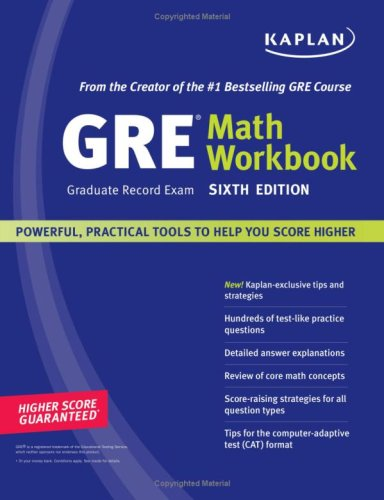# 6 Most-Tested GRE Problem Solving ConceptsNoticing that your scores on your GRE practice test isn’t quite as high as you’d like? One quick way to get better GRE Quantitative scores is to increase your content-knowledge in the most-tested Problem Solving areas. Here are the top seven most-tested GRE Quant concepts to review; get these down and you’ll ace the GRE section!

1. Functions and Symbols. A function is a different way of writing an equation. Instead of y = mx + b, we’d have f(x) = mx + b. It’s helpful to think of a function as simply replacing the “y” with a symbol called “f(x).” The GRE may also present made-up symbol functions; pay attention to any definitions you are given, and expand accordingly.

2. Number Properties. The properties of integers, primes, odds and evens, integers, fractions, positives, and negatives will all appear in various questions on your GRE test. The more comfortable you are with them, the more quickly you will arrive at the correct answer. This concept will bleed over into Quantitative Comparisons as well.

3. Plane and Coordinate Geometry. Not only will you need to know the standard equations for a line, parabola, and circle, but also you will need to memorize the distance formula, the midpoint formula, the slope formula, the relationship between slopes and the different quadrants, properties of parallel, perpendicular, vertical, and horizontal lines, as well as the quadratic formula/discriminant. For Plane Geometry, triangles are tested the most often on the GRE. You should know the Pythagorean Theorem, Triangle Inequality Theorem, the special right triangles: 45-45-90 and 30-60-90, as well as the properties of isosceles and equilateral triangles. Other plane geometry concepts to review include angles, circles, and polygons. Make sure you know how to find the perimeter and area of all shapes, and be comfortable dividing irregular shapes into manageable pieces.

4. Linear & Quadratic Equations. y = mx + b is the standard equation for a straight line, or a linear equation, where m is the slope and b is the y-intercept. You’ll need to know how to graph them and how to find the slope given two points. Quadratic equations look like y = ax2 + bx + c, and make a parabola, or curved line. Quadratics have two factors, and two solutions (also called “roots”). You will need to know how to factor quadratic equations to find the roots, how to find the quadratic if given the roots, and how to graph a quadratic on a grid given the equation.

5. Ratios and Proportions. A ratio is a relationship between two things. Given a ratio and one “real world” number, you can always set up a proportion to solve for the other missing “real world” number. Sometimes you will need to do this for similar triangles in Geometry, and sometimes in algebraic word problems.

6. Data Analysis. Data Analysis questions are like an open-book test. Make sure you read every tiny piece of writing on or near the data, including titles, the labels for the x and y-axes, column names, and even footnotes if there are any. Pay attention to the units of measurement, and notice any trends in the data BEFORE reading the questions.